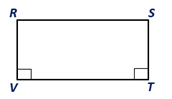Chapter 4.1, Problem 27E### Elementary Geometry for College St...

6th Edition
Daniel C. Alexander + 1 other
ISBN: 9781285195698

#### Solutions

Chapter
Section### Elementary Geometry for College St...

6th Edition
Daniel C. Alexander + 1 other
ISBN: 9781285195698
Textbook Problem
1 views

# In Exercises 27-30, use the definition of a parallelogram tocomplete each proof.Given: R S - ∥ V T - , R V - ⊥ V T - , and S T - ⊥ V T - Prove: RSTV is a parallelogramPROOF Statements Reasons 1. R S - ∥ V T - 1.? 2.? 2.Given 3.? 3.If two lines are ⊥ to the same line, they are ∥ , to each other 4.? 4.If both pairs of opposite side of a quadrilateral are ∥ , the quadrilateral is a ▱

To determine

To prove:

The quadrilateral RSTV is a parallelogram.

Explanation

Given:

In the quadrilateral RSTV, RS¯VT¯, RV¯VT¯, and ST¯VT¯.

 PROOF Statements Reasons 1. RS¯∥VT¯ 1.? 2.? 2.Given 3.? 3.If two lines are ⊥ to the same line, they are ∥, to each other 4.? 4.If both pairs of opposite side of a quadrilateral are ∥, the quadrilateral is a ▱

Corollary:

The opposite sides of a parallelogram are congruent.

From the given quadrilateral RSTV, RS¯VT¯, RV¯VT¯, and ST¯VT¯

### Still sussing out bartleby?

Check out a sample textbook solution.

See a sample solution

#### The Solution to Your Study Problems

Bartleby provides explanations to thousands of textbook problems written by our experts, many with advanced degrees!

Get Started

#### Find more solutions based on key concepts